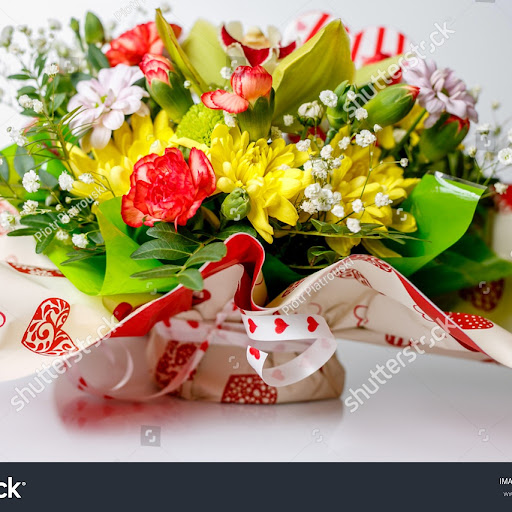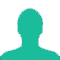# A shopkeeper earns a profit of 12% on selling a book at 10% discount on the printed price. The ratio of the cost price and the printed price of the book is:

A. 45 : 56

B. 45 : 51

C. 47 : 56

D. 47 : 51

### Solution(By Examveda Team)

\eqalign{ & {\text{Let}}\,{\text{the}}\,{\text{CP}}\,{\text{be}}\,100 \cr & {\text{Hence}}, \cr & {\text{SP}} = 100 + 12\% \,of\,100 \cr & \,\,\,\,\,\,\, = 112 \cr & {\text{If}}\,{\text{the}}\,{\text{marked}}\,{\text{price}}\,{\text{be}}\,X,\,{\text{then}} \cr & 90\% \,of\,X = 112 \cr & \Rightarrow x = \frac{{ {112 \times 100} }}{{90}} \cr & \Rightarrow x = Rs.\,\frac{{1120}}{9} \cr & {\text{Hence}}, \cr & {\text{Required}}\,{\text{ratio}} \cr & = 100:\,\frac{{1120}}{9} \cr & = 900:1120 \cr & = 45:56 \cr}

1.Other way solve it

2.112% of CP = 90% of MP
⇒ CP : MP = 45 : 56

3.a shopkeeper earns a profit of 155 after selling a books of 0% discount on the printed price. The ratio of the cost price and the printed price of the book is ?

4.@shivansh srivastava what you did was absolutely right
The ratio what you got is multiple of 46:56

5.LP*90%=CP*120%
CP:LP=3:4
is this wrong??
as here we have to find the ratio

6.a pencil together with an eraser cost rs. 3.80. if the cost of the pencil is rs. 1 more than that of the eraser, find the cost of eraser?

7.ritu had 400 mangoes.she sold 60 manoges at 25% gain,80 mangoes 20% gain,120 mangoes at gain 10% and 140 mangoes at 20% loss.what the net gain/loss percent??

plz tell me???

Related Questions on Profit and Loss